﻿ 基于Asmuth-Bloom门限共享秘密图像信息隐藏算法研究

# 基于Asmuth-Bloom门限共享秘密图像信息隐藏算法研究Research on Secret Image Threshold Sharing Information Hiding Algorithm Based on Asmuth-Bloom

Abstract: In order to transmit secret images more securely, this paper proposes a (t, n) threshold image data hiding algorithm based on Asmuth-Bloom secret sharing. First share the secret image into n meaningless secret shared images, use the DCT algorithm to embed the n meaningless images into n user-provided color cover images, and then distribute the n cover images with secret sharing to n users. Secondly, when the secret is recovered, users who are greater than or equal to the threshold t blindly extract meaningless secret sharing images from the cover image containing secret sharing, and then use the Chinese remainder theorem to recover the secret images. Finally, the experimental results proved that the algorithm proposed in this paper can recover the secret image correctly. This scheme is evaluated by peak signal-to-noise ratio (PSNR), mean structural similarity (MSSIM), and bit error ratio (BER), it is proved that this scheme is superior to other schemes.

1. 引言

2. 预备知识

2.1. 秘密共享技术

(1) 有任意t个或大于t个 ${s}_{i}$ 可以算出s。

(2) 若未拥有任意t个，只有任意t - 1个或更少份 ${s}_{i}$，不能算出s。

2.2. Asmuth-Bloom门限方案

Asmuth和Bloom基于中国剩余定理(CRT)提出了一个(t, n)门限方案，其中，成员们手中的共享份是由秘密s计算得到的y与设置的模数 ${m}_{1},{m}_{2},\cdots ,{m}_{n}$ 进行取模运算得到的 $\left({m}_{i},{y}_{i}\right)$

2.2.1. 参数选取

(1) $q>s$

(2) $\mathrm{gcd}\left({m}_{1},{m}_{j}\right)=1,\text{\hspace{0.17em}}\forall i,j,\text{\hspace{0.17em}}i\ne j$

(3) $\mathrm{gcd}\left(q,{m}_{i}\right)=1,\text{\hspace{0.17em}}i=1,2,\cdots ,n$

(4) $N={\prod }_{i=1}^{t}{m}_{i}>q{\prod }_{j=1}^{t-1}{m}_{n-j+1}$

2.2.2. 秘密共享

$\left({m}_{i},{y}_{i}\right)$ 即一个子共享份，将它分发给系统中的n个用户。

t个用户 ${i}_{1},{i}_{2},\cdots ,{i}_{t}$ 拿出自己的子份额时，由 $\left\{\left({m}_{{i}_{j}},{y}_{{i}_{j}}\right)|i=1,2,\cdots ,t\right\}$ 建立方程组

$\left\{\begin{array}{l}y\equiv {y}_{{i}_{1}}\mathrm{mod}{m}_{{i}_{1}}\\ y\equiv {y}_{{i}_{2}}\mathrm{mod}{m}_{{i}_{2}}\\ \text{\hspace{0.17em}}\text{\hspace{0.17em}}\text{\hspace{0.17em}}\text{\hspace{0.17em}}\text{\hspace{0.17em}}\text{\hspace{0.17em}}\text{\hspace{0.17em}}\text{\hspace{0.17em}}\text{\hspace{0.17em}}\text{\hspace{0.17em}}\text{\hspace{0.17em}}⋮\\ y\equiv {y}_{{i}_{t}}\mathrm{mod}{m}_{{i}_{t}}\end{array}$

$y\equiv {y}^{\prime }\mathrm{mod}{N}^{\prime }$

2.2.3. 秘密恢复

t个用户 ${i}_{1},{i}_{2},\cdots ,{i}_{t}$ 拿出自己的子份额时，由 $\left\{\left({m}_{{i}_{j}},{y}_{{i}_{j}}\right)|i=1,2,\cdots ,t\right\}$ 建立方程组

$\left\{\begin{array}{l}y\equiv {y}_{{i}_{1}}\mathrm{mod}{m}_{{i}_{1}}\\ y\equiv {y}_{{i}_{2}}\mathrm{mod}{m}_{{i}_{2}}\\ \text{\hspace{0.17em}}\text{\hspace{0.17em}}\text{\hspace{0.17em}}\text{\hspace{0.17em}}\text{\hspace{0.17em}}\text{\hspace{0.17em}}\text{\hspace{0.17em}}\text{\hspace{0.17em}}\text{\hspace{0.17em}}\text{\hspace{0.17em}}\text{\hspace{0.17em}}⋮\\ y\equiv {y}_{{i}_{t}}\mathrm{mod}{m}_{{i}_{t}}\end{array}$

$y\equiv {y}^{\prime }\mathrm{mod}{N}^{\prime }$

2.3. DCT信息隐藏算法嵌入参数选择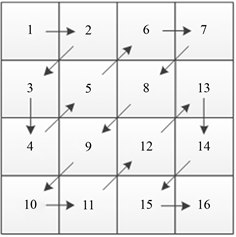Figure 1. Zigzag scanning sequence diagram

3. 基于Asmuth-Bloom门限秘密共享方案信息隐藏算法

3.1. 子秘密共享图像生成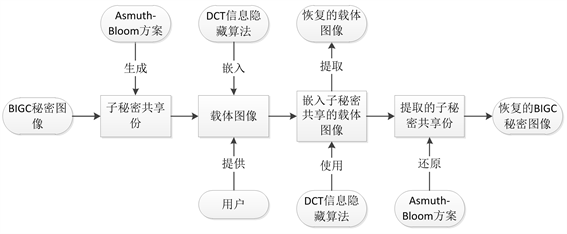Figure 2. Flow chart of the scheme

3.2. DCT信息隐藏算法

$\left\{\begin{array}{l}K{12}_{i}\ge 0\text{\hspace{0.17em}}\text{\hspace{0.17em}}\text{\hspace{0.17em}}\text{\hspace{0.17em}}{W}_{i}=1\\ K{12}_{i}<0\text{\hspace{0.17em}}\text{\hspace{0.17em}}\text{\hspace{0.17em}}\text{\hspace{0.17em}}{W}_{i}=0\end{array}$

${W}_{i}=0$ 时，保证DCT系数 $K{12}_{i}\le -k$，参数k是水印嵌入强度，嵌入公式如下：

$K{12}_{i}=\left\{\begin{array}{l}-K{12}_{i}\text{\hspace{0.17em}}\text{\hspace{0.17em}}\text{\hspace{0.17em}}\text{\hspace{0.17em}}\text{\hspace{0.17em}}\text{\hspace{0.17em}}\text{\hspace{0.17em}}K{12}_{i}>k\\ -k\text{\hspace{0.17em}}\text{\hspace{0.17em}}\text{\hspace{0.17em}}\text{\hspace{0.17em}}\text{\hspace{0.17em}}\text{\hspace{0.17em}}\text{\hspace{0.17em}}\text{\hspace{0.17em}}\text{\hspace{0.17em}}k\ge K{12}_{i}\ge -k\\ K{12}_{i}\text{\hspace{0.17em}}\text{\hspace{0.17em}}\text{\hspace{0.17em}}\text{\hspace{0.17em}}\text{\hspace{0.17em}}\text{\hspace{0.17em}}\text{\hspace{0.17em}}\text{\hspace{0.17em}}K{12}_{i}<-k\end{array}$

${W}_{i}=1$ 时，保证DCT系数 $K{12}_{i}\ge k$，嵌入公式如下：

$K{12}_{i}=\left\{\begin{array}{l}K{12}_{i}\text{\hspace{0.17em}}\text{\hspace{0.17em}}\text{\hspace{0.17em}}\text{\hspace{0.17em}}\text{\hspace{0.17em}}\text{\hspace{0.17em}}\text{\hspace{0.17em}}K{12}_{i}>k\\ k\text{\hspace{0.17em}}\text{\hspace{0.17em}}\text{\hspace{0.17em}}\text{\hspace{0.17em}}\text{\hspace{0.17em}}\text{\hspace{0.17em}}\text{\hspace{0.17em}}\text{\hspace{0.17em}}\text{\hspace{0.17em}}k\ge K{12}_{i}\ge -k\\ -K{12}_{i}\text{\hspace{0.17em}}\text{\hspace{0.17em}}\text{\hspace{0.17em}}\text{\hspace{0.17em}}\text{\hspace{0.17em}}K{12}_{i}<-k\end{array}$

${W}_{i}=\left\{\begin{array}{l}1\text{\hspace{0.17em}}\text{\hspace{0.17em}}\text{\hspace{0.17em}}\text{ }\text{ }K{12}_{i}\ge 0\\ 0\text{\hspace{0.17em}}\text{\hspace{0.17em}}\text{\hspace{0.17em}}K{12}_{i}<0\end{array}$

3.3. 秘密图像恢复

4. 实验结果

4.1. 基于(3, 5)门限秘密图像共享信息隐藏算法

$N={\prod }_{1}^{3}{m}_{i}=478661>q×{m}_{4}×{m}_{5}=7×89×97=60431$，参数A在 $\left[0,⌊\frac{N}{q}⌋-1\right]$ 范围内随机选取，即[0, 68379]

$\left\{\begin{array}{l}{y}_{1}\equiv y\mathrm{mod}{m}_{1}\equiv 361467\mathrm{mod}73\equiv 44\\ {y}_{2}\equiv y\mathrm{mod}{m}_{2}\equiv 361467\mathrm{mod}79\equiv 42\\ {y}_{3}\equiv y\mathrm{mod}{m}_{3}\equiv 361467\mathrm{mod}83\equiv 2\\ {y}_{4}\equiv y\mathrm{mod}{m}_{4}\equiv 361467\mathrm{mod}89\equiv 38\\ {y}_{5}\equiv y\mathrm{mod}{m}_{5}\equiv 361467\mathrm{mod}97\equiv 45\end{array}$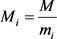，这是除了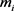以外其他两个模数的乘积。还需计算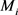意义下的逆元，公式表示为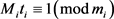。综合以上可以求出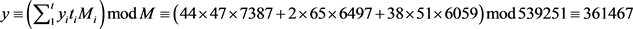。最后一步得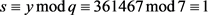4.2. (3, 5)门限信息隐藏实验结果分析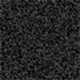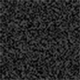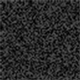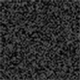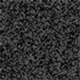Figure 3. The original image and the generated secret sharing image(a)(b)(c)(d)(e)(f)

Figure 4. Comparison of carrier image between Lena original image and embedded secret sharing imageTable 1. PSNR value and MSSIM value of this scheme are compared with other schemesFigure 5. The generated secret sharing image and the restored secret imageTable 2. BER value of image before and after restoration

5. 结语

 Shamir, A. (1979) How to Share a Secret. Communications of the ACM, 22, 612-613.
https://doi.org/10.1145/359168.359176

 Asmuth, C. and Bloom, J. (1983) A Modular Approach to Key Safe-guarding. IEEE Transactions on Information Theory, 29, 208-210.
https://doi.org/10.1109/TIT.1983.1056651

 王丽娜, 张焕国, 叶登攀, 等. 信息隐藏技术与应用[M]. 武汉: 武汉大学出版社, 2012: 17-18.

 Kutter, M., Jordna, F. and Bossen, F. (1997) Digital Signature of Color Images Using Amplitude Modulation. Proceedings Volume 3022, Storage and Retrieval for Image and Video Databases V, 200-205.
https://doi.org/10.1117/12.263442

 张亚泽. 图像秘密共享技术在信息保护中的应用研究[D]: [硕士学位论文]. 西安: 西安理工大学, 2020.

 Deng, Y., Kenney, C., Moore, M.S., et al. (1999) Peer Group Filtering and Perceptual Color Image Quantization. IEEE International Symposium on Circuits and Systems, Orlando, 30 May-2 June 1999, 21-24.

 唐明伟. 图像信息隐藏与隐藏分析算法研究[D]: [博士学位论文]. 成都: 电子科技大学, 2012.

 Yuan, H.D. (2014) Secret Sharing with Multi-Cover Adaptive Steganography. Information Sciences, 254, 197-212,
https://doi.org/10.1016/j.ins.2013.08.012

 Singh, P. and Raman, B. (2018) Reversible Data Hiding Based on Shamir’s Secret Sharing for Color Images over Cloud. Information Sciences, 422, 77-97.
https://doi.org/10.1016/j.ins.2017.08.077

Top